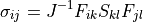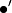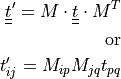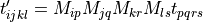# sfepy.mechanics.tensors module¶

Functions to compute some tensor-related quantities usual in continuum mechanics.

Encapsulates functions to convert various stress tensors in the symmetric storage given the deformation state.

get_cauchy_from_2pk(stress_in)[source]

Get the Cauchy stress given the second Piola-Kirchhoff stress.sfepy.mechanics.tensors.dim2sym(dim)[source]

Given the space dimension, return the symmetric storage size.

sfepy.mechanics.tensors.get_deviator(tensor, sym_storage=True)[source]

The deviatoric part (deviator) of a tensor.

sfepy.mechanics.tensors.get_full_indices(dim)[source]

The indices for converting the symmetric storage to the full storage.

sfepy.mechanics.tensors.get_non_diagonal_indices(dim)[source]

The non_diagonal indices for the full vector storage.

sfepy.mechanics.tensors.get_sym_indices(dim)[source]

The indices for converting the full storage to the symmetric storage.

sfepy.mechanics.tensors.get_t4_from_t2s(t2s)[source]

Get the full 4D tensor with major/minor symmetries from its 2D matrix representation.

Parameters
t2sarray

The symmetrically-stored tensor of shape (S, S), where S it the symmetric storage size.

Returns
t4array

The full 4D tensor of shape (D, D, D, D), where D is the space dimension.

sfepy.mechanics.tensors.get_trace(tensor, sym_storage=True)[source]

The trace of a tensor.

sfepy.mechanics.tensors.get_volumetric_tensor(tensor, sym_storage=True)[source]

The volumetric part of a tensor.

sfepy.mechanics.tensors.get_von_mises_stress(stress, sym_storage=True)[source]

Given a symmetric stress tensor, compute the von Mises stress (also known as Equivalent tensile stress).

Notessfepy.mechanics.tensors.prepare_cylindrical_transform(coors, origin, mode='axes')[source]

Prepare matrices for transforming tensors into cylindrical coordinates with the axis ‘z’ in a given origin.

Parameters
coorsarray

The Cartesian coordinates.

originarray of length 3

The origin.

mode‘axes’ or ‘data’

In ‘axes’ (default) mode the matrix transforms data to different coordinate system, while in ‘data’ mode the matrix transforms the data in the same coordinate system and is transpose of the matrix in the ‘axes’ mode.

Returns
mtxarray

The array of transformation matrices for each coordinate in coors.

sfepy.mechanics.tensors.sym2dim(sym)[source]

Given the symmetric storage size, return the space dimension.

Notes

This function works for any space dimension.

sfepy.mechanics.tensors.transform_data(data, coors=None, mode='cylindrical', mtx=None)[source]

Transform vector or tensor data components between orthogonal coordinate systems in 3D using transformation matrix, that should express rotation of the original coordinate system to the new system denoted bybelow.

For vectors:For second order tensors:For fourth order tensors:Parameters
dataarray, shape (num, n_r) or (num, n_r, n_c)

The vectors (n_r is 3) or tensors (symmetric storage, n_r is 6, n_c, if available, is 1 or 6) to be transformed.

coorsarray

The Cartesian coordinates of the data. Not needed when mtx argument is given.

modeone of [‘cylindrical’]

The requested coordinate system. Not needed when mtx argument is given.

mtxarray

The array of transformation matricesfor each data row.

Returns
new_dataarray

The transformed data.